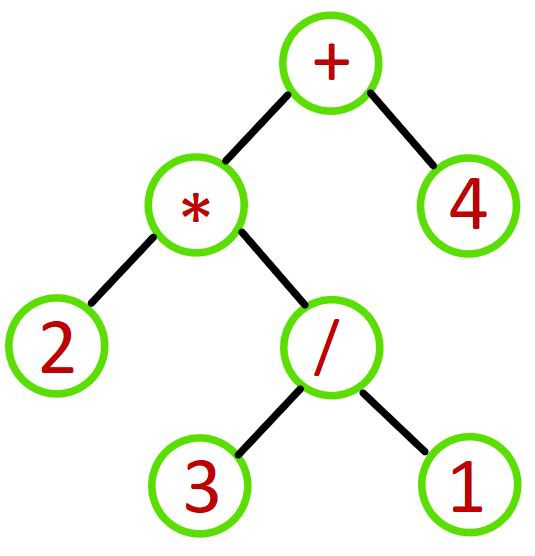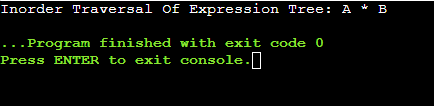# Expression Tree C++-Edited

Do you know what is expression tree C++? Have you ever thought to implement expression tree C++? Let us gain some knowledge about the process to implement an expression tree in C++.

But before we start to gather knowledge about expression tree in C++, let us first try to find out the method of the expression tree C++. For that purpose, let us assume one scenario from our daily life.Suppose, your friend provided you with a puzzle. You need to solve the puzzle. But your friend already solved the puzzle. Your task is to reseat the puzzle & again and complete the puzzle. So your initial task will be to destroy the completed puzzle. So, after destroying it what will you do?

You will keep those puzzle elements in one place. And one by one you will put them in this place. In this way, the puzzle gets solved. The same process happens in the expression tree C++. In this case, an expression is provided. Based on it, we need to make another form of expression.

Let us first try to know what expression tree C++ is. Then we will look into the expression tree example. This will help to clear our understanding.

What Is Expression Tree In C++:

An expression tree is a special kind of tree structure. We all know what is a tree in computer science. The tree is a type of Data Structure. There are many kinds of tree present. As per the function & structure of the tree, the category of the trees is changed. The expression tree is a binary tree. A binary tree is a type of tree where the maximum node allowed per root is two. This means, there can be a maximum of two leaves for each root node.

Read Also >>  A DIY Guide to Sync Google Calendar with Outlook

The same structure is followed by the expression tree. In the expression tree, there are some more limitations. Like in the expression tree, the variable will only be the leaf node of that tree. The variables or operands of any expression will be the leaf node. And the operators will be the root node. There are two types of expression trees. One is for the arithmetic expression. Another is for Boolean expression. But the arithmetic expressions are the most important & widely used.

Process To Create Expression Tree C++:

The theoretical approach of the expression tree is very unique. There we will use the tree concept & stack concept as well. Stack is another data structure. Stack has a First-In-Last-out approach. This means the element which will be inserted first, will be eliminated at the last. But here, we are not using the approach. We are here using the stack for scoring purposes. The elements of the expressions will store inside the stack. And whenever there will be an operator encountered, the operands will be popped out. Let us take one expression tree example.

Suppose, we are considering one postfix expression AB*. Here A and B are the operands or the variables.

1. Whenever the program will encounter any variable, it will simply store it in the stack. So, in the 1st step, the variables will store in the stack one by one.

2. In the 2nd step, it will encounter the operator. Whenever the program will encounter the operator, it will pop out the last entered stack elements. In this case, the last entered element was A & B. so, they will be popped out. And they will act as the left & right leaf nodes of the expression tree. The expression tree has the root node as the operator.

This will be a simple step. This step will be followed for every expression. Whenever there are variable or operand encounters, just we need to insert them inside the stack. Else, if we encounter the operator, we need to pop out two elements from the stack. One will be the left leaf node. Another will be the right leaf node. And the operator will become the root node. If there are many more elements still present, then the whole tree will be stored inside of the stack & the process again repeats.

Implementation Process Of Expression Tree C++:

For implementing the Expression Tree C++, we need to first create some basic things. Like we need to create a class that will act as the node. Also, we need to create another class that will act as the stack. A stack that can store nodes inside of it. Also, we need to create one function, that will help to pop out elements.

At first, we have taken one postfix expression there. Then from the expression, we are trying to find out the operator. If we encounter the operator, then two elements will be popped out from the stack. if there is no operator at the beginning, so the elements will be inserted into the stack.

After all of this operation, we need to use the Inorder of the expression tree. Inorder follows a default path. As we all know, it follows the rule LNR. This means the left node will print first, then the root node. And at last, the right node will be printed. In this way, the normal expression we will get. If you want to get C++ Homework Help then you can reach codingzap. They have the best coding experts in C++ and C Programming language.

Read Also >>  Top 10 React Naive Tools To Boost Your Speed of Mobile App Development

#include <bits/stdc++.h> using namespace std;

class node {public: char value; node* left; node* right; node* next = NULL;

node(char c){ this->value = c; left = NULL; right = NULL;} node(){ left = NULL; right = NULL;}

friend class Stack; friend class expression_tree; };

class Stack { node* head = NULL; public: void push(node*); node* pop(); friend class expression_tree; };

class expression_tree { // Class For Inorder Traversal

public: void inorder(node* x){

if (x == NULL) return; else { inorder(x->left); cout << x->value << ” “; inorder(x->right);}}};

void Stack::push(node* x) { // Function To Push Values

node* Stack::pop() { // Function To Implement Pop

int main(){string s = “AB*”; // Postfix ExpressionStack e; expression_tree a; node *x, *y, *z; int l = s.length();

for (int i = 0; i < l; i++) {

if (s[i] == ‘+’ || s[i] == ‘-‘|| s[i] == ‘*’ || s[i] == ‘/’|| s[i] == ‘^’) {

z = new node(s[i]); x = e.pop(); y = e.pop(); z->left = y; z->right = x; e.push(z);}

else { z = new node(s[i]); e.push(z); }}cout << “Inorder Traversal Of Expression Tree: “; a.inorder(z); return 0;}

Example:

Let us try to find out the output of the above code. This will help to understand the Expression Tree example.
Output:Conclusion:

As we saw Expression Tree C++ is a very important topic.
Expression tree in C++ helps a lot to solve difficult expression-related problems in the future. we also need to clear the expression tree example in a better way.
It is advisable to clear the basics of the C++ programing language. Also, we need to clear the theories related to the Data Structure & the Binary Tree Data Structures.